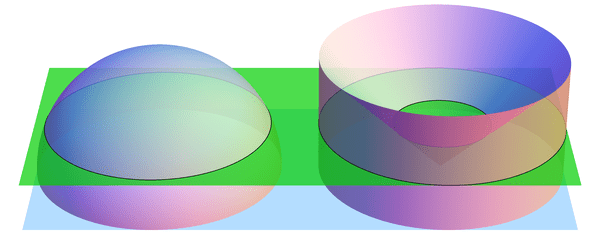# Slicing Spheres

Last week we saw how to compute the area of a circle from first principles. What about spheres?

To compute the volume of a sphere, let’s show that a hemisphere (with radius $$r$$) has the same volume as the vase shown in the figure below, formed by carving a cone from the circular cylinder with radius and height $$r$$. Why this shape? Here’s why: if we cut these two solids at any height $$h$$ (between 0 and $$r$$), the areas of the two slices match. Indeed, the slice—usually called cross section—of the sphere is a circle of radius $$\sqrt{r^2-h^2}$$, which has area $$\pi(r^2-h^2)$$. Similarly, the vase’s cross section is a radius $$r$$ circle with a radius $$h$$ circle cut out, so its area is $$\pi r^2-\pi h^2$$, as claimed.When sliced by a horizontal plane at any height $$h$$, the hemisphere and vase have equal cross-sectional areas. (Shown here for $$h = 0.4\cdot r$$.) By Cavalieri's Principle, this implies that they have equal volumes.

If we imagine the hemisphere and vase as being made from lots of tiny grains of sand, then we just showed, intuitively, that the two solids have the same number of grains of sand in every layer. So there should be the same number of grains in total, i.e., the volumes should match. This intuition is exactly right:

Cavalieri’s Principle: any two shapes that have matching horizontal cross sectional areas also have the same volume.

So the volumes are indeed equal, and all that’s left is to compute the volume of the vase. But we can do this! Recall that the cone has volume $$\frac{1}{3} (\text{area of base}) (\text{height}) = \frac{1}{3}\pi r^3$$ (better yet, prove this too! Hint: use Cavalieri’s Principle again to compare to a triangular pyramid). Likewise, the cylinder has volume $$(\text{area of base}) (\text{height}) = \pi r^3$$, so the vase (and hemisphere) have volume $$\pi r^3 – \frac{1}{3} \pi r^3 = \frac{2}{3}\pi r^3$$. The volume of the whole sphere is thus $$\frac{4}{3}\pi r^3$$. Success!

The following visualization illustrates what we have shown, namely $$\text{hemisphere} + \text{cone} = \text{cylinder}.$$ The “grains of sand” in the hemisphere are being displaced horizontally by the stabbing cone, and at the end we have exactly filled the cylinder.

## 3 thoughts on “Slicing Spheres”

1.Cameron Christensen says:

Your graphics are amazing! Would you mind sharing what program(s) you used to produce these images?

Thanks,

Cam

2.Cobie Howard says:

Hi,
I just found your website through a link on the British Interactive Group, a list by and for science communicators, based in the UK. Just a thought about your entry this week, “slicing Spheres:” the uninitiated (like me) might benefit from a one-sentence description, or a simple diagram, of what a “vase” is, early on in your entry. When I read “vase” and saw the diagram below it, I was completely baffled — I thought, “a vase, that’s a curvy hourglass shaped thing but with a rounded bottom, right?” On the other hand, reading further, I got it, enjoyed it, and will definitely keep looking at your posts. So no harm done. Thanks for your blog!
Cobie
P.S. My girlfriend is a Montessori-trained teacher of 4th-6th graders. Looking around her classroom one afternoon, I noticed a teaching material composed of a rectangular frame, an almost circular frame, (actually a 20-sided polygon) and a set of 20 triangles which can be used to fill either frame, so that kids can work through the proof you gave last week of the area of a circle. I thought, gee, that’s a neat thing, so it was gratifying to see on your blog that it has some legitimacy among real mathematicians as well. Do you think a 6th grader would benefit, either now or a few years hence, from a second and maybe third set of triangles of slightly different sizes, so they can get the idea of the two sums converging on pi, instead of the idea the material presents now, of the sum approaching from one direction only? That is, how important to the proof is it to come at the value of pi from both sides?
Thanks again!